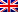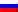# Divide and rule

Contenus

## Divide and rule

The method of divide and conquer is a method which allows, sometimes to find effective solutions to algorithmic problems. The idea is to cut out the initial problem, of size not, into several smaller sub-problems, then recombine the partial solutions until the final solution is obtained.

## Principle

Unlike dynamic programming, the results of the subproblems are only useful for the outcome of the parent problem.

The divide and conquer algorithm can be broken down into three steps:

1. Divide: the problem is split into size sub-problems b / w. These sub-problems are of the same nature as their parent. We are therefore in the presence of To identical subproblems. When b = 2, we are talking about dichotomy.
2. To rule: the sub-problems are solved recursively.
3. Recombine: the solutions of the subproblems are recombined to reconstruct the solution to the initial problem.

## Example

In order to better understand the construction of the algorithm, we will take the example of rapid exponentiation. The principle of this algorithm is to calculate xnot.

A naive method would be to do a loop where a * = x with a = 1 initially. The complexity of the naive method is therefore linear 0 (n).

In order to use the divide and conquer method, we need to find a mathematical program that allows us to rewrite our problem into equivalent subproblems. Since this is a recursive method, don't forget to include a final state. We are therefore in the presence of the following mathematical program:

The algorithm is as follows:

```double power (double x, int n) {
yew (n == 0) return 1;
else {
yew (n%2 == 0) return power (x * x, n / 2);
else return x * power (x * x, (n-1) / 2);
end if
end if
}```

Its complexity is 0 (log n) - size of the complete binary tree at not tops.FRFRENRUES